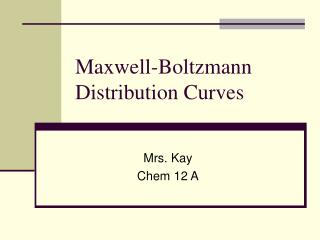Download PresentationMaxwell-Boltzmann Distribution Curves

Maxwell-Boltzmann Distribution Curves - PowerPoint PPT Presentation

Maxwell-Boltzmann Distribution Curves. Mrs. Kay Chem 12 A. In a sample gas or liquid, the molecules are in constant motion and collision. When they do this, no energy is lost from the system - they’re elastic collisions. Each molecule varies in its own energy, some have more, some less. .I am the owner, or an agent authorized to act on behalf of the owner, of the copyrighted work described.
Download PresentationMaxwell-Boltzmann Distribution Curves

Download Policy: Content on the Website is provided to you AS IS for your information and personal use and may not be sold / licensed / shared on other websites without getting consent from its author.While downloading, if for some reason you are not able to download a presentation, the publisher may have deleted the file from their server.

- - - - - - - - - - - - - - - - - - - - - - - - - - E N D - - - - - - - - - - - - - - - - - - - - - - - - - -
Presentation Transcript
1. Maxwell-Boltzmann Distribution Curves Mrs. Kay Chem 12 A

2. In a sample gas or liquid, the molecules are in constant motion and collision. • When they do this, no energy is lost from the system - they’re elastic collisions. • Each molecule varies in its own energy, some have more, some less.

3. A Maxwell-Boltzmann distribution curve/graph shows how the energy was spread out over different molecules

4. Remember… • the area under the curve = the total molecules in the sample, and it doesn’t change • All molecules have energy • Few molecules have high energies, but there is no maximum energy for a molecule.

5. If you increase the temperature of the sample, the distribution becomes stretched out and has a lower peak, but the area under the curve remains the same.

6. To speed up the reaction, you need to increase the number of the very energetic particles - those with energies equal to or greater than the activation energy. Increasing the temperature has exactly that effect - it changes the shape of the graph. / Energy

7. Molecularity and Rate Law

8. Molecularity • The number of molecules that participate as reactants in an elementary step • Unimolecular: a single molecule is involved. • Ex: CH3NC (can be rearranged) • Bimolecular: Involves the collision of two molecules • NO + O3 NO2 + O2 • Termolecular: simultaneous collision of three molecules. Far less probable.

9. Rate Law • Math expression to show how rate depends on concentration Rate = k[reactant 1]m[reactant 2]n… • m and n are called reaction orders. Their sum is called the overall reaction order. • k is the rate constant. It is specific to a reaction at a certain temperature. • The value of the exponents are determined experimentally, we cannot use stoichiometry!

10. Sample Exercise 1: 2NO + 2H2 N2 + 2H2O is the reaction we’re studying, this is the data found during our experimentation

11. Study the data Exp #1 and #2, [NO] is unchanged, [H2] is doubled and this causes the rate to double (0.0678/0.0339 =2), the H2’s rate order is 1. Exp #1 and #3, [H2] is unchanged, [NO] is doubled and this causes the rate to quadruple (0.1356/0.0339 =4), NO’s rate order is 2. Rate = k [NO]2[H2] The overall rate order for this reaction is 3 (1+2)

12. Suppose… If when we ran exp #1 and #3 , the rate didn’t change, what rate law would we expect? • Since it didn’t change when we doubled [NO], the rate order is 0, meaning the rate doesn’t depend on the concentration of NO at all, so rate= k[H2] and the overall reaction order is 1.

13. Sample Exercise 2: • Use the kinetics data to write the rate law for the reaction. What overall reaction order is this? 2NO + O22NO2

14. Sample Exercise 3 Rate data for the reaction: CH3Br + OH- CH3OH + Br- Use the data to find the experimental rate law.